# The frequency of rank 2 curves in a family of quadratic twists

Let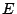be an elliptic curve over the rationals. Then its associated modular form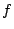is a newform of weight 2 with integral coefficients; let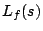be its associated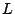-function. Suppose that the-function is scaled so that its functional equation relates the values ofat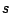and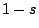. Suppose that the sign of the functional equation is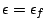and the level is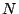. Let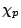be a real, primitive, Dirichlet character to the modulus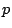,prime, for which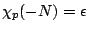so that the sign of the functional equation of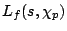is +1. We are interested in the number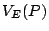of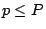for which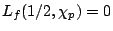. By the Birch and Swinnerton-Dyer conjecture, this will be the number of quadratic twists ofwith even rank at least 2. Goldfeld has conjectured that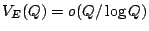for any.

In recent work of Conrey, Keating, Rubinstein, and Snaith [ arXiv:math/0012043] it was conjectured that there is a constant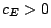such that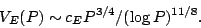The heuristics behind the conjecture involve applying random matrix theory to try to understand the value distribution of the-functions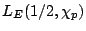and using the conjectural formula of Birch and Swinnerton-Dyer for this special value to determine how often the value is 0.

The method does not appear to give a value for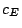. The reason seems to be that it is difficult to include in the reasoning the fact that one of the factors in the formula of Birch and Swinnerton-Dyer involves the order of a (finite) group, namely the Tate-Shafarevich group. The orders of random'' groups of size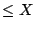, say, are not uniformly distributed over the interval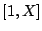, but instead depend on extra information, such as the automorphisms of the groups, as was observed in the work [ MR 85j:11144] of Cohen and Lenstra. It would be interesting to extend the Cohen-Lenstra heuristics to the present situation.

The problem, then, is to determine (heuristically) the constants. The method described above involves using a continuous distribution to conjecture how often a discrete quantity is zero. It is desirable to have a well-formulated approach for deciding in general how to turn a continuous distribution into a "cut off" function.

It would also be nice to have a geometric explanation for the exponents occurring in the above conjecture.

The above is inconsistent with a conjecture of Zagier and Kramarz [ MR 90d:11072]. They conjecture that a positive proportion of the curves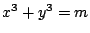have rank 2 or more, and they give both heuristic and numerical evidence for their conjecture. It can be argued that current numerical calculations are not able to distinguish between a constant and a power of the logarithm, rendering the numerics inconclusive. It is also possible that there is an interesting explanation (perhaps relating to small height points?) for why an excess of high rank seems to occur experimentally.

Back to the main index for L-functions and Random Matrix Theory.